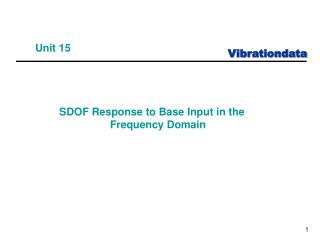DownloadDownload PresentationUnit 15

# Unit 15

Télécharger la présentation## Unit 15

- - - - - - - - - - - - - - - - - - - - - - - - - - - E N D - - - - - - - - - - - - - - - - - - - - - - - - - - -
##### Presentation Transcript

1. Unit 15 SDOF Response to Base Input in the Frequency Domain

2. Introduction • Steady-state response of an SDOF System • Base Inputs: Pure Sine PSD – stationary with normal distribution

3. SDOF System, Base Excitation The natural frequency fn is The damping coefficient C is The amplification factor Q is

4. SDOF Free Body Diagram The equation of motion was previously derived in Webinar 2.

5. Sine Transmissibility Function Either Laplace or Fourier transforms may be used to derive the steady state transmissibility function for the absolute response. After many steps, the resulting magnitude function is where where f is the base excitation frequency and fn is the natural frequency.

6. Frequency Ratio (f / fn) The base excitation frequency is f. The natural frequency is fn.

7. Transmissibility Curve Characteristics The transmissibility curves have several important features: 1. The response amplitude is independent of Q for f << fn. 2. The response is approximately equal to the input for f << fn. 3. Resonance occurs when f  fn. 4. The peak transmissibility is approximately equal to Q for f = fn and Q > 2. 5. The transmissibility ratio is 1.0 for f = 2 fn regardless of Q. 6. Isolation is achieved for f >> fn.

8. Exercises vibrationdata > Miscellaneous Functions > SDOF Response: Steady-State Sine Force or Acceleration Input Practice some sample calculations for the sine acceleration base input using your own parameters. Try resonant excitation and then +/- one octave separation between the excitation and natural frequencies. How does the response vary with Q for fn=100 Hz & f =141.4 Hz ?

9. “Better than Miles Equation” • Determine the response of a single-degree-of-freedom system subjected to base excitation, where the excitation is in the form of a power spectral density • The “Better than Miles Equation” is a.k.a. the “General Method”

10. Miles Equation & General Method • The Miles equation was given in a previous unit • Again, the Miles equation assumes that the base input is white noise, with a frequency content from 0 to infinity Hertz • Measured power spectral density functions, however, often contain distinct spectral peaks superimposed on broadband random noise • The Miles equation can produce erroneous results for these functions • This obstacle is overcome by the "general method" • The general method allows the base input power spectral density to vary with frequency • It then calculates the response at each frequency • The overall response is then calculated from the responses at the individual frequencies

11. General Method The general method thus gives a more accurate response value than the Miles equation. The base excitation frequency is fi and the natural frequency is fnThe base input PSD is

12. Navmat P-9492 Base Input PSD Overall Level = 6.06 GRMS Accel (G^2/Hz) Frequency (Hz)

13. Apply Navmat P-9492 as Base Input fn = 200 Hz, Q=10, duration = 60 sec Use: vibrationdata > power spectral density > SDOF Response to Base Input

14. SDOF Acceleration Response = 11.2 GRMS = 33.5 G 3-sigma = 49.9 G 4.47-sigma SDOF Pseudo Velocity Response = 3.42 inch/sec RMS = 10.2 inch/sec 3-sigma = 15.3 inch/sec 4.47-sigma SDOF Relative Displacement Response = 0.00272 inch RMS = 0.00816 inch 3-sigma = 0.0121 inch 4.47-sigma • 4.47-sigma is maximum expected peak from Rayleigh distribution • Miles equation also gives 11.2 GRMS for the response • Relative displacement is the key metric for circuit board fatigue per D. Steinberg (future webinar)

15. Pseudo Velocity • The "pseudo velocity" is an approximation of the relative velocity • The peak pseudo velocity PV is equal to the peak relative displacement Z multiplied by the angular natural frequency • Pseudo velocity is more important in shock analysis than for random vibration • Pseudo velocity is proportional to stress per H. Gaberson (future webinar topic) • MIL-STD-810E states that military-quality equipment does not tend to exhibit shock failures below a shock response spectrum velocity of 100 inches/sec (254 cm/sec) • Previous example had peak velocity of 15.3 inch/sec (4.47-sigma) for random vibration

16. Peak is ~ 100 x Input at 200 Hz Q^2 =100 Only works for SDOF system response Half-power bandwidth method is more reliable for determine Q.

17. Peak Design Levels for Equivalent Static Load

18. Rayleigh Peak Response Formula Consider a single-degree-of-freedom system with the index n. The maximum response can be estimated by the following equations. Maximum Peak a.k.a. crest factor

19. Conclusions • The General Method is better than the Miles equation because it allows the base input to vary with frequency • For SDOF System (fn=200 Hz, Q=10) subjected to NAVMAT base input… We obtained the same response results in the time domain in Webinar 14 using synthesized time history! • Response peaks may be higher than 3-sigma • High response peaks need to be accounted for in fatigue analyses (future webinar topic)

20. Homework • Repeat the exercises in the previous slides • Read T. Irvine, Equivalent Static Loads for Random Vibration, Rev N, Vibrationdata 2012 T. Irvine, The Steady-state Response of Single-degree-of-freedom System to a Harmonic Base Excitation, Vibrationdata, 2004 T. Irvine, The Steady-state Relative Displacement Response to Base Excitation, Vibrationdata, 2004